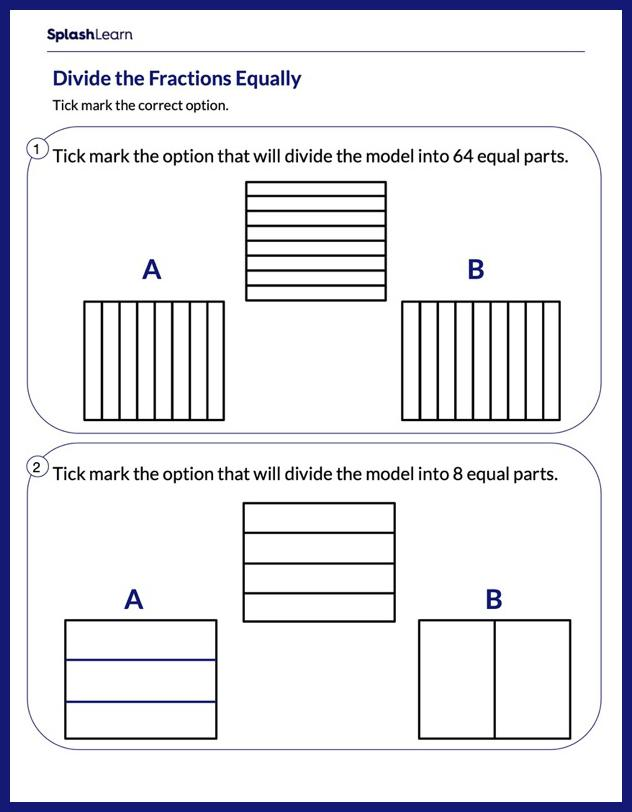# Divide the Model Equally Worksheet

Home > Divide the Model EquallyBuild your child's fraction skills with this worksheet. The worksheet is about dividing a shape into an equal number of parts by using fraction models as visual help. What better way is there for your young mathematician to learn than to have fun while learning? This worksheet will stimulate their minds and make learning more fun and lively!